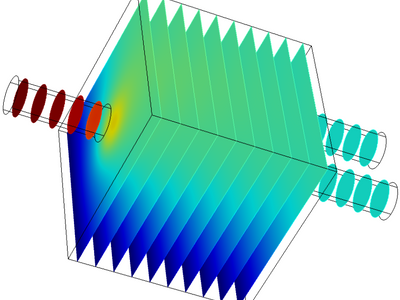SF2520 Applied Numerical Methods 7.5 creditsChoose semester and course offering

Choose semester and course offering to see information from the correct course syllabus and course offering.

Headings with content from the Course syllabus SF2520 (Autumn 2020–) are denoted with an asterisk ( )

Content and learning outcomes

Course contents

The course will give you knowledge about advanced computer methods based on numerical algorithms for solving mathematical models from scientific and engineering applications, in particular about how to formulate, analyze and implement them. More specifically, the course includes:

• numerical treatment of ordinary differential equations,
• finite difference methods and basic finite element methods for, mainly linear, partial differential equations,
• numerical solution of linear systems of equations by direct and iterative methods,

Intended learning outcomes

For the mathematical models in the course contents (e.g. ordinary and partial differential equations, linear systems of equations) the student shall be able to:

• select suitable numerical algorithms,
• analyze numerical methods with respect to computational cost, accuracy and stability,
• apply and implement numerical algorithms in a suitable programming language,
• classify and characterize the mathematical models.

In addition, the student shall be able to:

• estimate the accuracy of numerical results,
• describe limitations of mathematical models and numerical methods,
• for a given numerical problem, present, discuss and summarize the problem, solution method and results in a clear way,
• work inteams to solve a numerical problem.

Course disposition

No information inserted

Literature and preparations

Specific prerequisites

• Completed basic course in numerical analysis (SF1544, SF1545or equivalent)
• Completed basic course in differential equations (SF1633, SF1683or equivalent).

Recommended prerequisites

Completed basic courses in numerical analysis equivalent to SF1544 or similar, mathematical
courses corresponding to linear algebra, calculus and differential equations, good handling
with MATLAB.

Equipment

No information inserted

Literature

Lennart Edsberg: Introduction to computation and modeling for differential equations, Wiley 2008, ISBN 978-0-470-27085-1

Examination and completion

If the course is discontinued, students may request to be examined during the following two academic years.

A, B, C, D, E, FX, F

Examination

• LABA - Laboratory Work, 4.5 credits, grading scale: A, B, C, D, E, FX, F
• TEN1 - Written Examination, 3.0 credits, grading scale: A, B, C, D, E, FX, F

Based on recommendation from KTH’s coordinator for disabilities, the examiner will decide how to adapt an examination for students with documented disability.

The examiner may apply another examination format when re-examining individual students.

Opportunity to complete the requirements via supplementary examination

No information inserted

Opportunity to raise an approved grade via renewed examination

No information inserted

ExaminerOlof Runborg

Ethical approach

• All members of a group are responsible for the group's work.
• In any assessment, every student shall honestly disclose any help received and sources used.
• In an oral assessment, every student shall be able to present and answer questions about the entire assignment and solution.

Further information

Course web

Further information about the course can be found on the Course web at the link below. Information on the Course web will later be moved to this site.

Course web SF2520

SCI/Mathematics

Main field of study

Mathematics, Technology

Second cycle